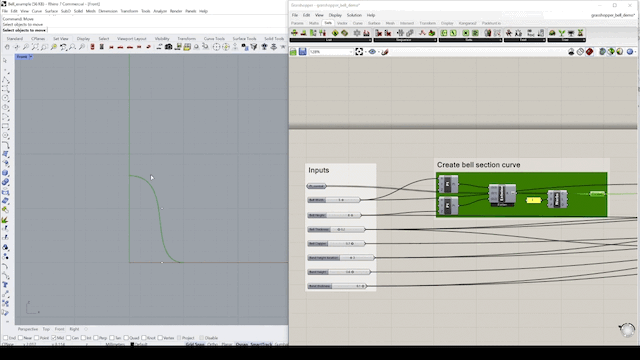top of page
Search

# 014 – Basic Grasshopper geometry

## Basic Geometry

Here, we generate a surface by using a curve & a circle. We use the sweep component to create a surface using the curve and following the circle. These are basic geometry types used in Grasshopper and are the building blocks to build more complex geometry.

Prerequisite: Different x,y,z coordinates in your graph. You can import them from your Rhino model as well. We will be using a bell geometry in this example.

## Steps:

Step 1: Turn the line into a curve by using control points. Moving the points in the Rhino model will influence the shape of the curve, the first two points will define the start and the end of the curve.Note: This means that the geometry can be influenced by parameters in the Grasshopper model as well as from the Rhino model.

Step 2: Here we generate a surface by using a curve and a circle. We use the 'sweep' component to create a surface using the curve to follow the circle.Note: A BREP is a collection of surfaces that together represent a boundary.These are the basic geometry types used in Grasshopper and are the building blocks to build more complex geometry.